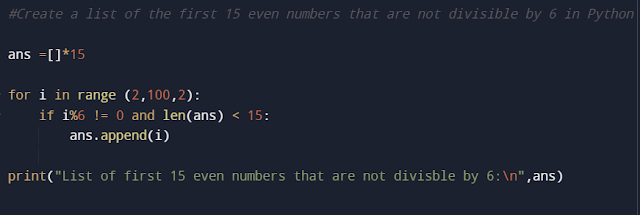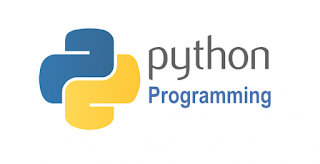## Pages

### Python program to Create a list of the first 15 even numbers that are not divisible by 6

Code:😃

ans =[]*15
for i in range (2,100,2):
if i%6 != 0 and len(ans) < 15:
ans.append(i)

print("List of first 15 even numbers that are not divisble by 6:\n",ans)Python
Output:🙌🙌

### Sample programs: Functions basics

Simple programs - solution for practice:

Copy and try on your own.
1) First 10 prime numbers program in python
code:
n = 10
count=0
print("First ten prime no program in Python")
for i in range(1,n+1):
for j in range(2,n+1):
if i % j == 0:
count+=1
if count == 1:
count=0

### Lists in Python

What is List?
• List is one of the most common collection datatypes in Python which is ordered and mutable (changeable).
• It is used to store a group of an element together in a sequence.
• List can store both homogenous and heterogeneous elements i.e., a single list may contain data types like integers, floats, strings as well as objects.
• Individual value in a list is called as elements.
• These elements will be stored in a contiguous memory location.
For Ex:  Number1 = 10
Number2 = 20
Number3 = 30,
Number4 = 40
• These individual variables can be represented in a list as
Number_list = [ 10, 20, 30, 40 ]

### Functions in Python basics

Functions In python:

• Set of instructions to perform a specific task.
• Below is the syntax of functions in python:
def function_name([arg1,....,arg n]):
statement 1
........
statement n
[return value]
variable = function_name([value1,......,value n])

### Control Structures

Control Structures in Python:
• In general, Control structures decide the flow of the program execution.
• The most commonly used control structures in python are
• Sequential: control flows through all the statements, in the order of how the code is written.
• Selection: Depends on the condition, control flows to the different statements.
• Iteration: Certain blocks of code(statements) will be executed repeatedly.

### Basics of Python

Basics of Python:

Python was created by Guido van Rossum in 1991 as a general-purpose programming language. It is interpreted and interactive language. Python is an open-source language under a general public license (GPL). It supports both object-oriented and structured style of programming.

### About: Introduction to Python Course

Introduction to Python:

This course is to introduce the basics of the Python programming language.Python was created by Guido van Rossum in 1991 as a general-purpose programming language. It is interpreted and interactive language. Python is an open-source language under a general public license (GPL). It supports both object-oriented and structured style of programming.

### Python Operators

Python Operators:
• Operators are special symbols that used to perform operations on the variables and the values.
• Consider an expression 10 + 20 = 30. Here, + is an operator that performs addition. 10 and 20 are called operands and 30 is the output of the operation.
• In general, the operand is a variable or a value that the operator operates on.
• Most commonly used operator groups in python language are listed below
• Arithmetic Operators
• Comparison/Relational Operators
• Logical Operators
• Assignment Operators
• Bitwise Operators
• Identity Operators
• Membership Operators
• Let's have a look at each operator with their syntax.

### AS400 Control Language (CL) Interview questions

In this blog post, we will see what are the major CL commands used in IBM iSeries/AS400. And what are the CL command questions asked during an interview. Let's see one by one below.